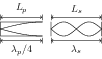# Vibration of Strings and Air Columns

## Problems from IIT JEE

Problem (IIT JEE 2010): A hollow pipe of length 0.8 m is closed at one end. At its open end a 0.5 m long uniform string is vibrating in its second harmonic and it resonates with the fundamental frequency of the pipe. If the tension in the string is 50 N and the speed of sound is 320 m/s, the mass of the string is,

1. 5 grams
2. 10 grams
3. 20 grams
4. 40 grams

Solution:The fundamental mode in a pipe closed at one end and the second harmonic in a string are shown in figure. It can be seen that $\lambda_p/4=L_p$ and $\lambda_s=L_s$. For the pipe closed at one end, \begin{align} \label{zda:eqn:1} \nu_p=\frac{v_p}{\lambda_p}=\frac{v_p}{4L_p}=\frac{320}{4(0.8)}=100\;\mathrm{Hz}, \end{align} where $v_p={320}\;\mathrm{m/s}$ is the velocity of sound in the pipe and $L_p={0.8}\;\mathrm{m}$ is length of the pipe. For string of mass $m$, length $L_s$ and having tension $T$, velocity of sound in the string is given by, \begin{align} \label{zda:eqn:2} \nu_s=\frac{v_s}{\lambda_s}=\frac{\sqrt{T/(m/L_s)}}{L_s}=\sqrt{\frac{T}{mL_s}}=\sqrt{\frac{50}{m(0.5)}}=\frac{10}{\sqrt{m}}. \end{align} At resonance, $\nu_p=\nu_s$. Substitute $\nu_p$ and $\nu_s$ from first and second equations to get $m={0.01}\;\mathrm{kg}={10}\;\mathrm{gram}$.

Problem (IIT JEE 2000): Two vibrating strings of the same material but of lengths $L$ and $2L$ have radii $2r$ and $r$ respectively. They are stretched under the same tension. Both the strings vibrate in their fundamental modes, the one of length $L$ with frequency $\nu_1$ and the other with frequency $\nu_2$. The ratio $\nu_1/\nu_2$ is given by,

1. $2$
2. $4$
3. $8$
4. $1$

Solution: Let the lengths and radii of the two strings be $l_1=L$, $r_1=2r$, $l_2=2L$, and $r_2=r$, respectively. Let $\rho$ be the density of the material. The mass and mass per unit length of the first string are, \begin{align} &m_1=\rho (\pi r_1^2 l_1)=4\pi \rho r^2 L,&& \label{dya:eqn:1} &\mu_1=m_1/l_1=4\pi \rho r^2, \end{align} and that of the second string are, \begin{align} &m_2=\rho (\pi r_2^2 l_2)=2\pi \rho r^2 L, && \label{dya:eqn:2} &\mu_2=m_2/l_2=\pi \rho r^2. \end{align} In fundamental mode, $l=\lambda/2=v/(2\nu)$ which gives, \begin{align} \label{dya:eqn:3} \nu=\frac{v}{2l}=\frac{1}{2l}\sqrt{\frac{T}{\mu}}. \end{align} Substitute $\mu$ from first and second equation to get, \begin{align} &\nu_1=\frac{1}{2l_1}\sqrt{\frac{T_1}{\mu_1}}=\frac{1}{2L}\sqrt{\frac{T}{4\pi\rho r^2}}=\frac{1}{4L}\sqrt{\frac{T}{\pi\rho r^2}},\nonumber\\ &\nu_2=\frac{1}{2l_2}\sqrt{\frac{T_2}{\mu_2}}=\frac{1}{2(2L)}\sqrt{\frac{T}{\pi\rho r^2}}=\frac{1}{4L}\sqrt{\frac{T}{\pi\rho r^2}}.\nonumber \end{align}